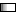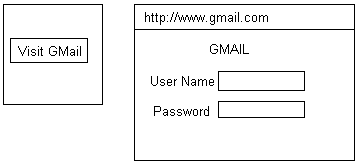Thursday, 2020-10-22, 12:04 PM
Tag Board
 --15 sec30 sec1 min2 min500Our poll How is .Net classes by R.N.Reddy sir ? Excellent Very Good Good Average Not Satisfied Total of answers: 242
 StatisticsTotal online: 1 Guests: 1 Users: 0
25-12-2010

Math(): Math is a predefined java script object, using this object we can perform mathematical operations

Example to implement round():

round(): It is a pre-defined member method of math object.

This method will return the nearest integral value of given float value.

Syntax: Math.round(<float value>)
Ex:   <html>
<body>
<script type = "text/javascript">
document.write(math.round(4.6) +"<br>")
document.write(math.round(-3.7) +"<br>")
document.write(math.round(1.2) +"<br>")
</script>
</body>
</html>
Output: 5
-4
1

Eg: for random method:

random(): This method will return a return a random number from 0 to 1
<html>
<body>
<script type = "text/javascript">
document.write(math.random() +"<br>")
document.write(math.random() +"<br>")
</script>
</body>
</html>
Output: 0.29
0.15

Eg: for maximum of two numbers

Max(): This method will return the maximum no. of two numbers.
<html>
<body>
<script type = "text/javascript">
document.write(math.max(10,5) +"<br>")
document.write(math.max(-1,-2) +"<br>")
document.write(math.max(-1,0) +"<br>")
</script>
</body>
</html>
Output: 10
-1
0

Eg: To find small (minimum no. of) given two numbers

Max(): This method will return the smallest no. of given two numbers.
<html>
<body>
<script type = "text/javascript">
document.write(math.min(10,5) +"<br>")
document.write(math.min(-1,-2) +"<br>")
document.write(math.min(-1,0) +"<br>")
</script>
</body>
</html>
Output: 5
-2
-1

Boolean Object: It is a java script build-in object it may represent with either true value or false value.

Eg: To create java script user defined object.

var myboolean = new Boolean()

Here, "myboolean" is a user defined object, Boolean() is a pre-efined object.
Java script boolean object will be representing with false value in below cases.

1. When it has not initialised.
2. When it has initialised with zero value.
3. When it has initialised with null value.
4. When it has initialised with false value.
5. When it has initialised with empty string.
6. When it has initialised with "-0".

Except the above cases java script boolen object will be representing with true value.

When the object is representing with null & false values with double quotes also will return true value because "null", and "full" will treate as user defined strings

Eg: To boolean Object:
<html>
<body>
<script type = "text/javascript">

var b1 = new boolean()
var b2 = new boolean(0)
var b3 = new boolean(null)
var b4 = new boolean(false)
var b5 = new boolean("")
var b6 = new boolean(-0)
var b7 = new boolean("Microsoft")
var b8 = new boolean(1)
var b9 = new boolean(true)
var b10 = new boolean(-1)
var b11 = new boolean("false")
var b12 = new boolean(4.5)

document.write(" No value is " +b1 +"<br>")
document.write("0 value is " +b2 +"<br>")
document.write("Null value is " +b3 +"<br>")
document.write("False value is " +b4 +"<br>")
document.write("Empty string value is " +b5 +"<br>")
document.write("-0 value is " +b6 +"<br>")
document.write("String value is " +b7 +"<br>")
document.write("1 value is " +b8 +"<br>")
document.write("True value is " +b9 +"<br>")
document.write("-1 value is " +b10 +"<br>")
document.write("False value is " +b11 +"<br>")
document.write("Float value is " +b12 +"<br>")

</script>
</body>
</html>
Output: No value is F
0 value is F
Null value is F
False value is F
Empty string value is F
-0 value is F
String value is T
1 value is T
False string value is T
Float value is T

Window Object: It is a pre-defined java script object. It holds complete information about the current web browser.

Using this object methods,  properties we can work on current web browser

Open(): Using this method we can open a web page based on given url.

Syntax: window.Open(<url>)

Requirment: for opening a new webpage<html>
<script type = "text/javscript">
function displaywindow()
{
window.open(http://wwww.gmail.com)
}
</script>
<body>
<input type = "Button" value = "visit Gmail" onclick= "display window()">
</body>
</html>

 Search

 Time

Calendar

 «  October 2020  » Su Mo Tu We Th Fr Sa 1 2 3 4 5 6 7 8 9 10 11 12 13 14 15 16 17 18 19 20 21 22 23 24 25 26 27 28 29 30 31